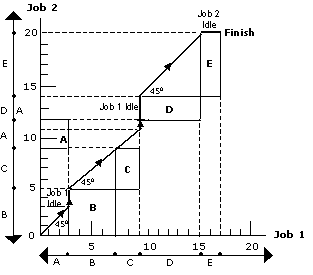# Two jobs and m – Machines Problems

This section focuses on the sequencing problem of processing two jobs through m machines. Problems under this category can be solved with the help of graphical method. The graphical method below is explained with the help of the following example.

#### Example

Two jobs are to be performed on five machines A, B, C, D, and E. Processing times are given in the following table.

 Job 1 Machine Sequence : A B C D E Time : 3 4 2 6 2 Job 2 Sequence : B C A D E Time : 5 4 3 2 6

Use graphical method to obtain the total minimum elapsed time.

### Solution.

#### Steps

• Mark the processing times of job 1 & job 2 on X-axis & Y-axis respectively.
• Draw the rectangular blocks by pairing the same machines as shown in the following figure.• Starting from origin O, move through the 450 line until a point marked finish is obtained.
• The elapsed time can be calculated by adding the idle time for either job to the processing time for that job. In this illustration, idle time for job 1 is 5 (3+2) hours.
Elapsed time = Processing time of job 1 + Idle time of job 1
= (3 + 4 + 2 + 6 + 2) + 5 = 17 + 5 = 22 hours.
Likewise, idle time for job 2 is 2 hours.
Elapsed time = Processing time of job 2 + Idle time of job 2
= (5 + 4 + 3 + 2 + 6) + (2) = 20 + 2 = 22 hours.

## One thought on “Two jobs and m – Machines Problems”

error: Content is protected !!